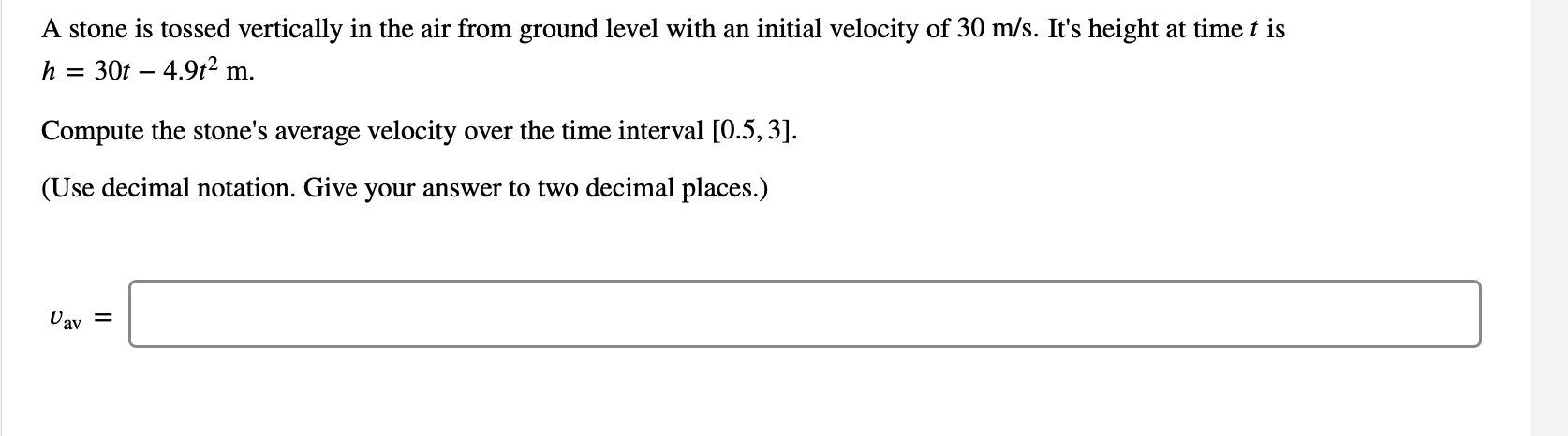# A stone is tossed vertically in the air from ground level with an initial velocity of 30 m/s. It's height at time t is30t 4.92 mhCompute the stone's average velocity over the time interval [0.5,3].(Use decimal notation. Give your answer to two decimal places.)Uav

Question
1 viewshelp_outlineImage TranscriptioncloseA stone is tossed vertically in the air from ground level with an initial velocity of 30 m/s. It's height at time t is 30t 4.92 m h Compute the stone's average velocity over the time interval [0.5,3]. (Use decimal notation. Give your answer to two decimal places.) Uav fullscreen
check_circle

Step 1

Given,

Initial Velocity = 30 m/s

Height of a stone at time t,

Step 2

Average velocity is given by

Step 3

continue...

### Want to see the full answer?

See Solution

#### Want to see this answer and more?

Solutions are written by subject experts who are available 24/7. Questions are typically answered within 1 hour.*

See Solution
*Response times may vary by subject and question.
Tagged in

### Derivative